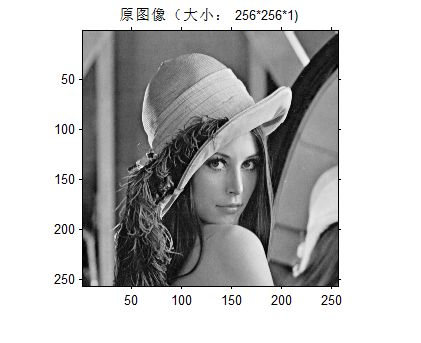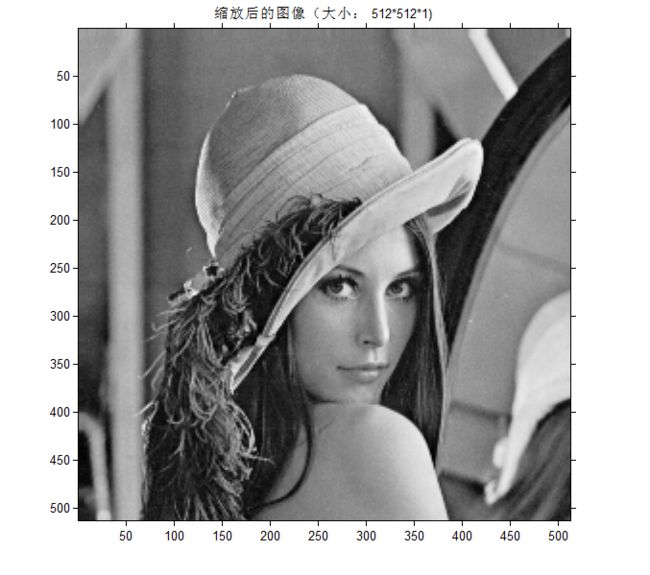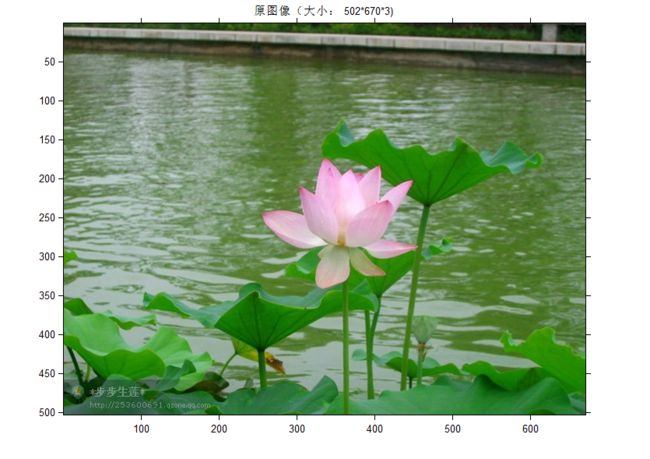• 有用，最好看看，双线性函数用的挺广的，想学点就下来看看什么的
• 矩阵分析与多元统计II 二次型与二次曲面2 双线性函数双线性函数双线性函数的表示双线性函数的简单性质矩阵的合同关系满秩双线性函数对称与反对称对称双线性函数反对称双线性函数应用伪正交变换辛变换对称双线性函数...
矩阵分析与多元统计II 二次型与二次曲面2 双线性函数双线性函数双线性函数的表示满秩双线性函数对称与反对称对称双线性函数反对称双线性函数应用伪欧氏空间与伪正交变换辛空间与辛变换对称双线性函数空间与反对称双线性函数空间
双线性函数
假设$V$是数域$F$上的线性空间，映射$f:V\times V \to F$若满足

$f(k_1\alpha_1+k_2\alpha_2,\beta)=k_1f(\alpha_1,\beta)+k_2f(\alpha_2,\beta),\forall \alpha_1,\alpha_2,\beta \in V, k_1,k_2 \in F$
$f(\alpha,c_1\beta_1+c_2\beta_2)=c_1f(\alpha,\beta_1)+c_2f(\alpha,\beta_2),\forall \alpha,\beta_1,\beta_2 \in V,c_1,c_2 \in F$

则称$f$是$V$上的一个双线性函数。
双线性函数的表示
假设$(e_1,e_2,\cdots,e_n)$是$V$的一组基，定义双线性函数$f$的度量矩阵为
$G = \left[ \begin{matrix} f(e_1,e_1) & f(e_1,e_2) & \cdots & f(e_1,e_n) \\ f(e_2,e_1) & f(e_2,e_2) & \cdots & f(e_2,e_n) \\ \cdots & \cdots & \cdots & \cdots \\ f(e_n,e_1) & f(e_n,e_2) & \cdots & f(e_n,e_n) \end{matrix} \right]$
给定基下，度量矩阵与双线性函数是一一对应的关系。与线性函数类似，假设$\alpha,\beta$在$(e_1,e_2,\cdots,e_n)$下的坐标是$x,y$，则
$f(a,b)=x'Gy$
假设$(\eta_1,\eta_2,\cdots,\eta_n)$是$V$的另一组基，从$(e_1,e_2,\cdots,e_n)$到$(\eta_1,\eta_2,\cdots,\eta_n)$的转移矩阵是$T$，即
$(\eta_1,\eta_2,\cdots,\eta_n) = (e_1,e_2,\cdots,e_n)T$
则
$G(f;\eta_1,\eta_2,\cdots,\eta_n)=T'G(f;e_1,e_2,\cdots,e_n)T$
也就是说同一个双线性函数在不同基下的度量矩阵是合同的。
满秩双线性函数
如果$f(\alpha,\beta)=0,\forall \beta \in V \Rightarrow \alpha=0$，则称$f$是满秩的，下面是满秩的等价性叙述：

$G$满秩；
$f(\alpha,\beta)=0,\forall \alpha \in V \Rightarrow \beta=0$

我们称定义了满秩双线性函数的线性空间为双线性度量空间，需要注意的是双线性度量空间不一定是度量空间，但满秩双线性函数的作用与度量非常类似，都是把两个向量映射到一个数值，所以给它一个名字叫双线性度量。
对称与反对称
假设$f(\alpha,\beta)=f(\beta,\alpha)$，则称$f$为对称双线性函数；假设$f(\alpha,\beta)=-f(\beta,\alpha)$，则称$f$为反对称双线性函数。
对称双线性函数
关于对称双线性函数，我们有一个非常有趣的例子。欧氏空间中的内积就是一个满秩的对称双线性函数，根据Schmidt正交化定理，在任何标准正交基下，内积的度量矩阵是单位矩阵。这给我们提供了一个有趣的视角来理解对称双线性函数，我们可以把对称双线性函数理解为欧氏内积的某种推广，称定义了满秩对称双线性函数的实线性空间为伪欧氏空间，比如用来描述四维时空的Minkowski空间在Minkowski内积下成为伪欧氏空间。另外，我们知道实对称矩阵可以对角化，因此存在一组基使得对称双线性函数度量矩阵成为对角阵。
基于一个一般的双线性函数$g$，我们可以定义对称双线性函数：
$f(\alpha,\beta) = \frac{g(\alpha,\beta)+g(\beta,\alpha)}{2}$
关于对称双线性函数，我们还想关注它与二次齐次函数的关系。对于$f(\alpha,\beta)$，当$\alpha=\beta$时，称$f(\alpha,\alpha)$是$f(\alpha,\beta)$对应的二次齐次函数。二次齐次函数与双线性函数是一一对应关系：
$f(\alpha,\beta)=\frac{f(\alpha+\beta,\alpha+\beta)-f(\alpha,\alpha)-f(\beta,\beta)}{2}$
反对称双线性函数
反对称双线性函数在给定基时可以用反对称矩阵表示，我们知道反对称矩阵是可以对角化为：
$diag(S_2,\cdots,S_2,0,\cdots,0),S_2=\left[ \begin{matrix} 0 & 1 \\ -1 & 0\end{matrix} \right]$
的形式的，因此存在一组基使得反对称双线性函数的度量矩阵满足这个形式。称定义了满秩反对称双线性函数的实线性空间为辛空间。
应用
伪欧氏空间与伪正交变换
在$n$维伪欧氏空间$V$中，满秩对称双线性函数在基$(e_1,e_2,\cdots,e_n)$下的度量矩阵满足$G=diag(I_p,-I_{n-p}),0 \le p\le n$
就称$(e_1,e_2,\cdots,e_n)$为标准正交基。如果$\mathcal{A}:V \to V$满足
$f(\mathcal{A}\alpha,\mathcal{A}\beta)=f(\alpha,\beta)$
就称$\mathcal{A}$是伪正交变换，假设$A$是$\mathcal{A}$的矩阵表示，称$A$是伪正交矩阵。关于伪正交变换有几个性质：

伪正交变换的逆是伪正交变换；
伪正交变换的积是伪正交变换；
$|A|=1\ or\ -1$
$A'GA=G$

我们可以从伪正交矩阵出发，假设$\alpha,\beta$在标准正交基$(e_1,e_2,\cdots,e_n)$下的坐标为$x,y$，则
$f(\alpha,\beta) =x'Gy$
对$\alpha,\beta$做伪正交变换后，它们的坐标变为$Ax,Ay$，因此
$f(\mathcal{A}\alpha,\mathcal{A}\beta) =x'A'GAy$
根据定义
$f(\mathcal{A}\alpha,\mathcal{A}\beta) = f(\alpha,\beta) \Rightarrow A'GA=G$
基于这个结果，我们可以计算行列式
$|A'GA|=(-1)^{n-p}=(-1)^{n-p}|A|^2 \Rightarrow |A|=1\ or\ -1$
另外，根据$A'GA=G$，我们知道$A$时满秩的，因此伪正交变换是满秩的变换，用$\mathcal{A}^{-1}$表示它的逆变换，则根据定义
$f(\mathcal{A}^{-1}\mathcal{A}\alpha,\mathcal{A}^{-1}\mathcal{A}\beta)=f(\alpha,\beta) = f(\mathcal{A}\alpha,\mathcal{A}\beta)$
因此伪正交变换的逆变换也是伪正交变换。假设$\mathcal{B}$是另一个伪正交变换，则根据定义
$f(\mathcal{B}\mathcal{A}\alpha,\mathcal{B}\mathcal{A}\beta)= f(\mathcal{A}\alpha,\mathcal{A}\beta)=f(\alpha,\beta)$
因此伪正交变换的积$\mathcal{B}\mathcal{A}$也是正交变换。
辛空间与辛变换
$V$是$2m$维的辛空间，$f$是$V$上的满秩反对称双线性函数，称$(e_1,e_2,\cdots,e_{2m})$为辛基，如果
$G = \left[ \begin{matrix} 0 & I_m \\ -I_m & 0 \end{matrix} \right]$
如果$\mathcal{A}:V \to V$满足
$f(\mathcal{A}\alpha,\mathcal{A}\beta)=f(\alpha,\beta)$
就称$\mathcal{A}$是辛变换。假设$A$是$\mathcal{A}$的矩阵表示，称$A$是辛矩阵。辛变换有下面几个性质，这几个性质的推导与伪正交变换基本一致：

辛变换的逆是辛变换；
辛变换的积是辛变换；
$A'GA=G$

对称双线性函数空间与反对称双线性函数空间
$V$是数域$F$上的线性空间，对称实双线性函数空间与反对称实双线性函数空间有几个结论：

$(V \times V)^*$是$n^2$维线性空间；
用$Sym(V \times V)^*$与$Alt(V \times V)^*$表示$(V \times V)^*$的满足对称、反对称性质的子集，证明二者的维数分别为$n(n+1)/2$, $n(n-1)/2$

上一讲介绍过线性空间的对偶空间与线性空间维数相同，因此
$dim(V \times V)^* = dim(V \times V)=dim(V) \times dim(V)=n^2$
下面考虑$Sym(V \times V)^*$与$Alt(V \times V)^*$的维数。结论非常直观，因为$n \times n$的对称矩阵有$n(n+1)/2$个自由度，反对称矩阵有$n(n-1)/2$个自由度。因此$Sym(V \times V)^*$与$Alt(V \times V)^*$的维数分别为$n(n+1)/2$, $n(n-1)/2$。


展开全文线性代数 机器学习
• 实验程序源代码 imblizoom.m文件源码 function [ ZI ] = imblizoom( I,zmf ) %----------------------双线性插值法缩放矩阵或图像--------------------------- % Input： % I：图像文件名或矩阵(整数值(0~255)) %...
三、实现1.  实验平台与数据本算法使用Matlab语言实现，实验平台为Windows 8 32位操作系统、4GB内存(可用为2.31GB)、Matlab2013b。数据1： 大小为：256*256 的lena灰度图像，将使用实现的算法对其进行2倍放大操作，如下图1所示：图1 灰度图像lena.png数据2：大小为：670*502 的彩色RGB图像，将使用实现的算法对其进行2倍缩小操作，如下图1所示：图2彩色RGB图像he.jpeg2.  实验程序源代码imblizoom.m文件源码function [ ZI ] = imblizoom( I,zmf )%----------------------双线性插值法缩放矩阵或图像---------------------------% Input：%       I：图像文件名或矩阵(整数值(0~255))%       zmf：缩放因子，即缩放的倍数% Output：%缩放后的图像矩阵ZI% Usage:%       ZI = SSELMHSIC('ImageFileName',zmf)%对图像I进行zmf倍的缩放并显示%    Or:%       ZI = SSELMHSIC(I,zmf)%对矩阵I进行zmf倍的缩放并显示%    ...%-------------------------------------------------------------------%%%%    Authors:   Zhi Liu%%%%    XiDian University Student%%%%    EMAIL:     zhiliu.mind@gmail.com%%%%    DATE:      16-12-2013%% Step1对数据进行预处理if ~exist('I','var') || isempty(I)error('输入图像I未定义或为空！');endif ~exist('zmf','var') || isempty(zmf) || numel(zmf) ~= 1error('位移矢量zmf未定义或为空或zmf中的元素超过2！');endif isstr(I)[I,M] = imread(I);endif zmf <= 0error('缩放倍数zmf的值应该大于0！');end%% Step2通过原始图像和缩放因子得到新图像的大小，并创建新图像。[IH,IW,ID] = size(I);ZIH = round(IH*zmf); %计算缩放后的图像高度，最近取整ZIW = round(IW*zmf); %计算缩放后的图像宽度，最近取整ZI = zeros(ZIH,ZIW,ID); %创建新图像%% Step3扩展矩阵I边缘IT = zeros(IH+2,IW+2,ID);IT(2:IH+1,2:IW+1,:) = I;IT(1,2:IW+1,:)=I(1,:,:);IT(IH+2,2:IW+1,:)=I(IH,:,:);IT(2:IH+1,1,:)=I(:,1,:);IT(2:IH+1,IW+2,:)=I(:,IW,:);IT(1,1,:) = I(1,1,:);IT(1,IW+2,:) = I(1,IW,:);IT(IH+2,1,:) = I(IH,1,:);IT(IH+2,IW+2,:) = I(IH,IW,:);%% Step4由新图像的某个像素(zi，zj)映射到原始图像(ii，jj)处，并插值。for zj = 1:ZIW         %对图像进行按列逐元素扫描for zi = 1:ZIHii = (zi-1)/zmf; jj = (zj-1)/zmf;i = floor(ii); j = floor(jj); %向下取整u = ii - i; v = jj - j;i = i + 1; j = j + 1;ZI(zi,zj,:) = (1-u)*(1-v)*IT(i,j,:) +(1-u)*v*IT(i,j+1,:)...+ u*(1-v)*IT(i+1,j,:) +u*v*IT(i+1,j+1,:);endendZI = uint8(ZI);%%以图像的形式显示同现矩阵Pfigureimshow(I,M);axis ontitle(['原图像(大小：',num2str(IH),'*',num2str(IW),'*',num2str(ID),')']);figureimshow(ZI,M);axis ontitle(['缩放后的图像(大小：',num2str(ZIH),'*',num2str(ZIW),'*',num2str(ID)',')']);end3.  实验结果1) 数据1在命令窗口输入imblizoom('lena.png', 2);回车后结果如下图3和下图4所示：图3 lena.png的运行结果1(原图)图4 lena.png的运行结果2(放大2倍后的图)2)数据2图5 he.jpeg运行结果1(原图)图6 he.jpeg运行结果2(缩小2倍后的图像)由图3~图6可知，程序正确无误，放大缩小后的效果都很好。程序的用法及其它功能，请查看代码注释。
展开全文• 一.双线性函数(10.1) 1.概念 (1)定义: (2)示例: 2.双线性函数的表达式 (1)度量矩阵的概念: (2)双线性型: (3)不同基下的度量矩阵间的关系:一.双线性函数(10.1)
1.概念
(1)定义:(2)示例:2.双线性函数的表达式
(1)度量矩阵的概念:(2)双线性型:(3)不同基下的度量矩阵间的关系:

①已知2个度量矩阵,求其关系:
定理1:设$f$是域$F$上$n$维线性空间$V$上的1个双线性函数,$V$中取2个基$α_1...α_n$与$β_1...β_n$,设$(β_1...β_n)=(α_1...α_n)P\qquad(6)$$f$在基$α_1...α_n$和基$β_1...β_n$下的度量矩阵分别为$A,B$,则$B=P'AP\qquad(7)$注:2种证明均可

②已知2个合同的矩阵,求其关系:
定理1’:如果$A\simeq B$,则$A,B$可看成是$V$上同1个双线性函数$f$在$V$的不同基下的度量矩阵*(4)双线性函数的矩阵秩:二.特殊的双线性函数(10.1)
1.非退化的双线性函数
(1)双线性函数的左/右根:(2)非退化的双线性函数:定理2:域$F$上的$n$维线性空间$V$上的双线性函数$f$是非退化的,当且仅当$f$在$V$的1个基下的度量矩阵是满秩矩阵2.(斜)对称双线性函数
(1)定义:(2)充要条件:

设$f$是域$F$上的$n$维线性空间$V$上的1个双线性函数,$f$在$V$的1个基$α_1...α_n$下的度量矩阵为$A$,则$f是对称的\qquad\qquad\qquad\qquad\qquad\\⇔f(α_i,α_j)=f(α_j,α_i)\quad i,j=1,2...n\\⇔A(i;j)=A(j;i)\qquad\:\:\:\:\: i,j=1,2...n\\⇔A是对称矩阵\qquad\qquad\qquad\qquad\qquad$类似地,有$\:\:\:\:f是斜对称的\qquad\qquad\qquad\qquad\qquad\\\,\,\,⇔f(α_i,α_j)=-f(α_j,α_i)\quad i,j=1,2...n\\⇔A(i;j)=-A(j;i)\qquad\,\,\: i,j=1,2...n\\\:\:\:\,\,⇔A是斜对称矩阵\qquad\qquad\qquad\qquad\qquad\:$

(3)(斜)对称双线性函数的度量矩阵的最简单的形式:定理3:设$f$是特征不为2的域$F$上的$n$维线性空间$V$上的对称双线性函数,则$V$中$∃1$个基,使得$f$在此基下的度量矩阵为对角矩阵推论1:特征不为$2$的域$F$上的$n$阶对称矩阵$A$一定合同于1个对角矩阵,这个对角矩阵称为$A$的1个合同标准型定理4:设$f$是特征不为2的域$F$上的$n$维线性空间$V$上的斜对称双线性函数,则$∃V$的$1$个基,记成$δ_1,δ_{-1}...δ_r,δ_{-r},η_1...η_s\,(0≤r≤\frac{n}{2},s=n-2r)$,使得$f$在此基下的度量矩阵具有如下形式:$diag\{\left[\begin{matrix}0&1\\-1&0\end{matrix}\right]...\left[\begin{matrix}0&1\\-1&0\end{matrix}\right],0...0\}\qquad(16)$推论1:特征不为$2$的域$F$上的$n$阶斜对称矩阵$A$一定合同于1个形如下式的分块对角矩阵:$\left[\begin{matrix}\left[\begin{matrix}0&1\\-1&0\end{matrix}\right]\\&...\\&&\left[\begin{matrix}0&1\\-1&0\end{matrix}\right]\\&&&0\\&&&&...\\&&&&&0\end{matrix}\right]$

注:特征为2的域$F$上的$n$维线性空间$V$上的对称双线性函数的度量矩阵的最简单的形式见附录

三.对称双线性函数与二次型的关系(10.1)
四.双线性函数空间(10.1)
附录.特征为2的域$F$上的$n$维线性空间$V$上的对称双线性函数的度量矩阵的最简单的形式


展开全文线性空间
• Abstract 超平面散列（Hyperplane hashing）的目的是快速搜索到离超平面最近的点，并在使用支持向量机（SVM）扩大主动学习方面显示出实际效果。...其核心思想是该散列函数双线性形式，在使用随机投影时比现有的超平面
Abstract
超平面散列（Hyperplane hashing）的目的是快速搜索到离超平面最近的点，并在使用支持向量机（SVM）扩大主动学习方面显示出实际效果。
存在问题：不幸的是，现有的随机方法需要长哈希码才能达到合理的搜索精度，因此会降低搜索速度和内存开销。
解决方法：为此，论文（Compact Hyperplane Hashing with Bilinear Functions）提出了一种新的超平面哈希技术，它可以产生紧凑的哈希码。其核心思想是该散列函数的双线性形式，在使用随机投影时比现有的超平面散列函数具有更高的碰撞概率。
为了进一步提高性能，我们提出了一个基于学习的框架，其中双线性函数直接从数据中学习。这将产生短而有区别的代码，并且比基于随机投影的解决方案提高了搜索性能。在两个数据集上进行的大规模主动学习实验证明了该方法的优越性。
1. Introduction
由于数据的大量增长，快速近似最近邻搜索（approximate nearest neighbor search）在各种领域和应用中普遍出现。克服速度瓶颈的有吸引力的解决方案。
彻底的线性扫描所带来的是使用局部敏感哈希（LSH）家族的算法（Gionis等人，1999）（Charikar，2002）（Datar等人，2004），这些算法使用随机投影将输入数据转换为二进制散列码。虽然在次线性散列/搜索时间和返回邻域的准确性方面有理论上的保证，但LSH相关的方法通常需要较长的代码和大量的代码和哈希表的数量才可以达到很好的搜索精度。这可能会导致相当大的存储开销和降低搜索速度。因此，在文献中，直接学习依赖于数据的散列函数来生成紧凑代码已成为一种流行。这种散列通常每个数据项需要少量的位，并且可以设计成使用单个散列表和恒定的散列时间。最先进的技术包括无监督哈希（Liu et al.，2011）、半监督哈希（Wang et al.，2012）和监督哈希（Liu et al.，2012）。
现有的散列方法大多试图解决点到点最近邻搜索问题。也就是说，查询和数据库项都被表示为某个特征空间中的单独点。
考虑到现实世界数据的复杂结构，过去还提出了点对点搜索之外的其他形式的散列范式，例如子空间到子空间最近邻搜索（Basri等人，2011）。
在这篇文章中，我们提出了一个更具挑战性的超平面搜索问题，其中查询在Rd中是超平面，即（d-1）维子空间，而数据库项是常规的点。那么搜索问题是：给定一个超平面查询和一个点数据库，返回到超平面距离最小的点。在文献中，关于点到超平面问题的研究还不多，但（Jain等人，2010）证明了这一问题在使基于SVM的主动学习在海量数据池上可行方面的重要性。
主动学习（AL）也称为基于池的主动学习，它通过选择几个样本进行标记来规避盲目标记的高成本。在每一次迭代中，典型的学习者从未标记的样本池中寻找信息量最大的样本，从而在标记选定样本后获得最大的信息增益。然后，在增量标记样本集上重新训练学习模型。经典算法（Tong&Koller，2001）使用支持向量机（SVM）作为学习模型。基于“版本空间”理论（Tong&Koller，2001），它证明了在对称版本空间（version spaces）假设成立的情况下，选择最接近当前决策超平面的样本。不幸的是，主动选择方法在应用于大型数据库时面临严重的计算挑战。寻找最佳样本的穷举搜索（exhaustive search ）通常在计算上是禁止的。因此，快速的点到超平面搜索被强烈地期望在大的实际数据集上扩大主动学习。
最近，在（Jain等人，2010）中提出了超平面哈希方案来处理点到超平面搜索。与通过所有数据库点的暴力扫描相比，这些方案在理论上保证了次线性查询时间和检索到的近似近邻的精度损失，显著提高了效率。因此，当对
支持向量机主动学习的样本选择任务，可以扫描数量级较少的数据库点来传递下一个主动标记请求，从而使主动学习具有可扩展性。
在（Jain et al.，2010）中，随机散列的两个家族证明了函数对角度的局部敏感性在数据库点和超平面查询之间；然而，长哈希位和大量哈希表必须满足理论保证。实际上，它采用了300位和500个表（Jain等人，2010）为了实现合理的绩效，这给计算和存储都带来了沉重的负担。
为了缓解上述问题，本文提出了一种紧凑的超平面哈希方案它只利用一个包含多个数十个哈希位来处理指向超平面搜索的问题。
我们的哈希方案的主旨是设计和学习双线性哈希函数，使得几乎平行的输入向量被哈希到相同的比特，而几乎垂直的输入向量被哈希到不同的比特。事实上，我们首先证明，即使没有任何学习，所提出的双线性的随机版本
与现有的方法相比，哈希方法具有更高的近邻居碰撞概率。图1 支持向量机主动学习中的点到超平面搜索问题。Pw是支持向量机的超平面决策边界，w是Pw的法向量，x是一个数据向量。（a） 点到超平面距离D（x，Pw）和指向超平面角αx，w；（b）提供有用信息的（x1，x2）和信息不足的（x3，x4）样本。
接下来，我们将双线性投影投射到一个学习框架中，并说明使用学习的散列函数可以做得更好。给定一个超平面查询，它的法向量作为输入，通过将学习到的哈希函数的输出位串联起来得到相应的哈希码。
然后，检索其代码与查询代码汉明距离最远的数据库点。关键的是，根据我们的学习原则，被称为接近超平面邻居的点与超平面保持小角度。在两个高达100万的大数据集上进行的实验证实了我们的方法能够以良好的性能实现可伸缩的主动学习。最后，虽然本文选择支持向量机SVM主动学习作为超平面散列的实验平台，但我们要强调的是，所提出的紧凑超平面散列方法是一种通用的方法适用于大范围的机器学习问题，如最小切线距离追踪和基于割平面的最大边缘聚类。
2. Problem
点到超平面的距离：the point-to-hyperplane angle：角度指标αx，w∈[0，π/2]
在数据库点和超平面之间的查询可以很容易地反映在哈希中。
如图1（b）所示，超平面哈希的目标是将超平面查询Pw和具有窄αx、w的信息样本（例如x1、x2）散列到相同或附近的散列桶中，同时避免返回宽度为αx，w的无信息样本（例如x3、x4），因为，点到超平面搜索问题可以等价地转化为一个特定的点对点搜索问题，其中查询是超平面法向量w，与原始查询Pw的期望最近邻是离w的角度θx最接近π/2的，即几乎与w相垂直。这与传统的点对点最近邻搜索有很大不同，后者到查询点的最相似的点。如果我们把作为x和w之间的相似性度量，则超平面散列实际上寻找满足| cos（θx∗，w）|→0中与查询点w最不相似的点x *。相反，最相似的点，如w或-w，对于主动选择准则来说肯定是不具信息性的，必须排除在外。
3. Randomized Hyperplane Hashing
在本节中，我们首先简要回顾现有的基于线性函数的随机哈希方法，然后
提出了我们的双线性随机散列方法，并对其进行了理论分析提出了双线性哈希函数。
3.1. Background – Linear Hash Functions
Jain等人。（Jain et al.，2010）设计了两种不同的随机散列函数族来解决超平面散列问题。
第一个是角超平面哈希（AH Hash） 其中一个例子是其中z∈Rd表示输入向量，u和v都是独立于独立于标准的双变量高斯函数，即u，v∼N（0，Id×d）绘制的。请注意hA是一个两位散列函数，它导致超平面法向w和a的碰撞概率：第二种是嵌入超平面哈希（EH Hash）。函数族ε的一个例子是EH还具有角度敏感散列属性。
然而，它比AH Hash要昂贵得多。
需要注意的是AH Hash和EH Hash本质上都是线性哈希技术。
相反，在这项工作中，我们引入了双线性哈希函数(bilinear hash functions)，它允许非线性哈希。
3.2. Bilinear Hash Functions
我们提出了一个双线性哈希函数，如下所示其中u，v∈Rd是两个投影向量。我们设计这种双线性形式的动机来自
以下两个要求：1）h对z应该有尺度不变性，这是由z和βz（β=0）具有相同的点到超平面角；2）h应为两个垂直输入向量生成不同的哈希位。由于双线性公式，前者肯定成立。我们在引理1中证明了当u，v与标准正态分布无关时，后者（等号后）具有恒定概率。图2.三种随机化方法的理论比较哈希方案。（a） p1（碰撞概率）与r（平方 点到超平面角）；（b）ρ（查询时间指数）与r，对于ε=3。
为了达到上述超散列平面的目的，双线性哈希函数的关键作用是映射查询点w（超平面法线）以及最理想的信息点（‘θx，w’ =π/2）到按位的不同的哈希码（hash code）;
而映射w和最不受欢迎的最不具信息性的指向相同的哈希代码。（有’θx，w’=0或π）。因此，超平面散列的工作原理是找到X中的点对于查询编码w具有最大的汉明距离。
3.3. Theoretic Analysis
介绍随机函数族BH-Hash双线性超平面哈希：有趣的是，AH散列和我们提出的BH散列在散列数据库点的风格上有着紧密的联系。BH-hash实际上对AH Hash输出的两个位执行XNOR（同或门；异或非门）操作，返回一个复合的单个位。作为相关参考，在构造散列函数时，在二进制位上应用XOR(异或)运算的思想曾在（Li&Konig，2010）中使用过。但是，这只适用于有限的数据类型，离散集，仍然属于点对点搜索。
4. Compact Hyperplane Hashing
尽管提出的BH-Hash比AH-Hash和EH-Hash具有更高的碰撞概率，但它仍然是一种随机方法。在hB中使用随机投影有两个潜在的问题。
（i） 平行Pw和x与αx，w=0碰撞的概率不太高（根据引理1，只有1/2）。（ii）散列时间为次线性O（nρlog1/p2n），以限制检索到的邻域的近似误差，如定理2所示。AH Hash和EH Hash也受到这两个问题的影响。尽管这些随机超平面散列方法保持有界的近似误差，但它们需要长的哈希码和大量（甚至数百个）哈希表来满足精度保证。因此，这些解决方案具有巨大的计算和内存开销，限制了超平面哈希的实际性能。
为此，我们提出了一种紧凑的超平面哈希方法，以进一步增强双线性散列函数的能力，使得投影不再是随机的，而是从数据中学习的。
这样的学习产生紧凑但有区别的代码，这些代码用于单个哈希表中，从而大大减少了计算和存储需求。
**我们的目标：**学习一系列双线性散列函数 {hj} 来生成短代码。注意hj不同于随机双线性散列函数hBj，我们一致地定义hj（Pw）= -hj（w）。我们想学习hj，这样αx，w越小，hj（Pw）hj（x）越大。因此，我们使hj（Pw）hj（x）随着αx，w的增加而单调减小。这相当于hj（w）hj（x）随sin（αx，w）=| cos（θx，w）|单调增加的要求。
学习目标：因此，所提出的学习方法实现了平行*Pw和x的显式碰撞。
执行式（11）倾向于使hj为几乎平行的输入生成相同的哈希码，而对于几乎垂直的输入，则产生按位不同的哈希码。在查询时，给定一个超平面查询，我们首先使用应用于超平面法向量的k个学习散列函数，提取其k位散列码。然后，返回其编码与查询编码的汉明距离最大的数据库点。因此，返回的点，称为接近超平面的邻居，与超平面保持小角度，因为这些点和超平面法线几乎垂直。
在我们的学习环境中，k通常很短，不超过30，因此我们可以通过对单个哈希表的恒定时间哈希来检索所需的近超平面邻居。
现在我们描述如何学习k对的投影：，从而构造k个双线性散列函数。
由于超平面法向量只在查询期间出现，我们在训练阶段无法访问w（法向量）。取而代之的是，我们对一些数据库点进行抽样以进行学习预测。在不损失一般性的前提下，我们假设存储在矩阵Xm=[x1，···，Xm]中的前m个（k<m≪n）样本用于学习。为了捕捉它们之间的成对关系，我们定义了一个矩阵S∈Rm×m为利用式（11）中给出的学习目标，我们构造了一个最小平方式的目标函数Q来学习Xm的二进制码B = [H⊤(x1), · · · , H⊤(xm)]⊤代表了矩阵Xm， ||.||F 表示Frobenius范数。
式（12）中使用的阈值t1，t2具有重要的
角色。当两个输入容易平行时从而**| cos(θxi,xi′ )|** 足够大（超过t1），使Q驱动每个位的编码发生碰撞，即H(xi)H⊤(xi′ )/k = 1;；当两个输入趋于垂直时，| cos(θxi,xi′ )| 足够小（小于t2），最小化Q试图使它们的代码按位不同，即H(xi)H⊤(xi′ )/k = -1。
用简单的代数，我们可以把Q重写为B=[b1，····，bk]中的每个位向量bj∈{1，-1}m 决定一个被一个投影对（uj，vj）参数化的散列函数hj。注意，bj在求和中是可分离的，这激发了一个贪婪的想法
按顺序求解bj。在给定先前求解的向量的情况下，它只涉及求解一个比特向量bj（uj，vj）. 定义一个剩余矩阵。然后，可以通过最小化以下成本来实现bj最终代价：注意g（uj，vj）是下界的，因为等式（14）总是非负的。然而，最小化g并不容易因为它既不凸也不光滑。在我们下面提出了一种近似优化算法。
因为最小化g的困难在于符号， we replace sgn() in bj with the sigmoid shaped function ϕ(x) = 2/(1 + exp((-x)) -1 which
is sufficiently smooth and well approximates sgn(x) when |x| > 6.
随后，我们提出优化一个对g的光滑代理surrogate ˜g哈达玛积最终优化的双线性哈希函数如下所示：不过，不像随机哈希方法，很难证明其理论性质，如碰撞概率，它们比随机函数hBj更精确，如随后的实验所证明的那样。
利用学习到的散列函数H=[h1，···，hk]，我们可以通过简单地将-1位处理为0位来实现所提出的紧凑超平面哈希。在预处理阶段，每个数据库点x被转换成k位散列码H（x），并以k位散列键作为条目存储在单个散列表中。为了在查询时进行搜索，在给定超平面法向量w的情况下，我们1）提取其哈希键H（w）并进行逐位NOT运算以获得密钥2）在哈希表中查找取NOT 中最近的项，直到达到很小的汉明距离，得到一个短列表L，L中其点是从找到的哈希桶中检索出来；3） 扫描列表L，然后返回点：事实上，在一个以翻转码为中心的小Hamming球内搜索，相当于在Hamming空间中搜索与H（w）码具有最大可能汉明距离的码。
5. Experiments
5.1. Datasets
我们在两个公开的网站上进行实验数据集包括20个新闻组文本语料库和8000万个微型图像集合中的106万个子集，称为Tiny-1M。第一个数据集是20个新闻组的22版。它是由来自20个新闻组类别的18846个文档。每个文档都用26214维表示归一化的tf-idf特征向量。
Tiny-1M数据集是CIFAR-10和从整个80M微型图像集采集的一百万个微小图像的结合。CIFAR-10是80M微型图像集的一个子集，由来自10个对象类别的60000个彩色图像组成，每个类别有6000个样本。其他1M图像没有带注释的标签。在我们的实验中，我们将它们作为CIFAR-10中出现的10个类之外的“另一个”类，因为它们被采样为离CIFAR-10平均图像的最远1百万个图像。Tiny-1M中的每幅图像都由一个384维的GIST来表示（Oliva&Torralba，2001）特征向量。
对于每个数据集，我们在一对所有设置（one-versus-all setting）中训练一个线性支持向量机linear SVM，初始标记集包含从所有类中随机选择的标记样本，然后运行活动样本选择300次迭代。最初标记为20个新闻组的集合包括每个类5个样本，而对于Tiny-1M，每个类包含50个样本。对于这两个数据集，我们尝试5个随机初始化。每个样本选择完成后，我们将其添加到标记的集合中，并重新训练SVM支持向量机。我们使用LIBLINEAR4运行linearSVMs.全部我们的实验是在一个有2.53ghz英特尔至强处理器和48GB内存的工作站上进行的。
5.2. Evaluations and Results
我们使用基于最小边距的样本选择准则进行支持向量机主动学习应用超平面哈希技术加快
选择程序。为了验证所讨论的超平面哈希方法的实际性能，我们将它们与两个基线进行比较：随机选择下一个标签请求是随机的，以及对所有当前未标记样本评估裕度标准的穷举选择。我们比较四种散列方法，包括两种随机线性散列方案AH-Hash和EH-Hash（Jain等人，2010），提出的随机双线性散列方案BH-Hash，以及我们称之为基于学习的双线性哈希方案LBH哈希。请注意，我们对AH Hash、BH Hash和LBH Hash的初始化使用了相同的随机投影来阐明双线性散列（XNOR两位）的效果。我们也遵循维度抽样技巧（Jain et al.，2010）加速EH Hash的计算。
为了训练我们提出的LBH哈希，我们随机抽取500个样本以及来自20个新闻组和分别是Tiny-1M。使用的两个阈值t1，t2。为了实现显式碰撞，根据以下规则得到：
compute the absolute cosine matrix C between the m sampled points {xi} and all data, average the top 5% values among Ci. across
xi’s as t1, and average the bottom 5% values as t2.
为了使散列方法在一个紧凑的哈希模式下工作，以便进行公平比较，我们使用了一个
具有短代码长度的单个哈希表。具体来说，我们为EH hash、BH hash和LBH - hash使用16个哈希位，AH hash使用32位，因为它在20个新闻组上具有双位哈希精神。在AL迭代中应用每个哈希方法时，我们执行在相应的哈希表中，在Hamming radius 3范围内进行哈希查找，然后扫描所找到的哈希桶中的点，从而使邻居接近当前支持向量机的决策超平面。同样，我们在Tiny-1M上，EH Hash、BH Hash和LBH Hash使用20位，AH Hash使用40位；搜索的Hamming radius设置为4。一个方法有可能在Hamming ball中找到所有空的散列桶。在这种情况下，我们应用随机选择作为补充。
我们从以下几个方面评估了四种散列方法的性能：1）在每次AL迭代中，用当前SVM分类器对当前未标记样本集进行排序，计算出平均精度（AP）；2） 每次AL迭代时超平面散列返回的邻居的最小边距（最小点到超平面距离；3）对于接收非空哈希查找的每个类，总共300个查询中的查询数。前两个结果在所有类和5次运行中取平均值，后一个结果在5次运行中取平均值。我们在图3和图4中报告了这些结果，它们清楚地表明
1） LBH Hash达到最高平均AP（MAP）在所有比较过的散列方法中，甚至在某些迭代中优于穷举选择；
2）LBH Hash通过穷举选择实现最接近该值的最小边距；
3）LBH Hash几乎享受所有非空哈希查找（AH Hash几乎得到所有空查找）。LBH-Hash的优越性能证明了所提出的双线性Hash函数和相关的学习技术能够成功地利用底层数据信息生成紧凑而有区别的代码。
最后，我们在补充材料的表1-3中报告了计算效率，结果表明LBH-Hash与EH-Hash具有相当的预处理时间，并获得了较快的搜索速度。6. Conclusions
我们通过提出一个专门的双线性哈希函数，允许高效
搜索超平面查询附近的点。即使什么时候
利用随机投影，提出了哈希函数，与现有的随机方法相比，碰撞概率更高。通过学习预测
此外，我们实现了紧凑而有区别的代码，这使得在存储和搜索过程中需要的时间。在两个数据集上的大规模主动学习实验证明了我们的紧凑超平面的优越性能


展开全文机器学习
• 用双线性函数的语言：数域K上n维线性空间V内任一对称双线性函数的矩阵都可对角化；即，V内存在一组基，使该对称双线性函数在此组基下的矩阵成对角形矩阵。 用矩阵论的语言：数域K上任一对称的n阶方阵都合同于一个对...高等代数
• 双线性插值法原理：① 何为线性插值？插值就是在两个数之间插入一个数，线性插值原理图如下：在位置 x 进行线性插值，插入的值为f(x) ↑② 各种插值法：插值法的第一步都是相同的，计算目标图(dstImage)的坐标点...
• #coding=utf-8import cv2import numpy as np'''双线性插值'''img = cv2.imread('timg.jpeg', cv2.CV_LOAD_IMAGE_GRAYSCALE) # load the gray imagecv2.imwrite('img.jpg', img)h, w = img.shape[:2]# shr...
• 1，原理在图像的仿射变换中，很多地方需要用到插值运算，常见的插值运算包括最邻近插值，双线性插值，双三次插值，兰索思插值等方法，OpenCV提供了很多方法，其中，双线性插值由于折中的插值效果和运算速度，运用...
• 运用双线性插值函数可以将图像的离散表示近似转化为一个连续函数双线性插值函数是一个连续的曲面 z=y(xg11+(1-x)g01)+(1-y)(xg10+(1-x)g00)  g00, g01, g10, g11是相邻四个像素点的灰度值  这里可以令：  g...图像处理
• 这里有一个可重用的函数。它包括文档测试和数据验证：def bilinear_interpolation(x, y, points):'''Interpolate (x,y) from values associated with four points.The four points are a list of four triplets: (x,...
• import numpy as npimport cv2##双线性插值def resize(src,dstsize):#输入src 和sizeif src.ndim==3:dstsize.append(3)dst=np.array(np.zeros(dstsize),src.dtype)factory=float(np.size(src,0))/dstsizefactorx=...
• 基本插值函数def interpolation(y0,x0, y1,x1, x):frac = (x - x0) / (x1 - x0)return y0*(1-frac) + y1 * frac步骤1：将原始坐标映射到新调整尺寸的图像def get_coords(im, W, H):h,w = im.shapex = np.arang...
• 实现OpenCV的双线性插值函数，分享给大家共同学习。特别注意以下几点： 1、取整运算 直接使用int强制转换时截断取整，加上一个0.5矫正系数变为四舍五入取整 2、分段移位 猜测是因为cbufy 为short类型，必须将dx...opencv
• 我发现了很多关于这个主题和许多答案的...此函数可以将列表作为x和y坐标，并且无需循环即可执行查找和求和。def bilinear_interpolate(im, x, y):x = np.asarray(x)y = np.asarray(y)x0 = np.floor(x).astype(int)x...
• 用fortan方法编写的双线性插值算法函数，可以用其他语言仿照编写，共参考
• 总结了大半年，控制系统各种传递函数双线性变换离散化后的递推公式。相信能帮助大家
• 双线性内插值： 对于一个目的像素，设置坐标通过反向变换得到的浮点坐标为(i+u,j+v)， 其中i、j均为非负整数，u、v为[0,1)区间的浮点数，则这个像素得值 f(i+u,j+v) 可由原图像中坐标为 (i,j)、(i+1,j)、(i,j+1)、...
• 图像处理技术的基础函数双线性差值，在图像不失真的情况下，进行缩放，经测试完成。可顺利运行。
• 自己编的可放大任意整数倍的最近邻插值 双线性插值 matlab代码
• 双线性系统非线性传递函数矩阵的计算公式，对于mimo的双线性系统，计算其传递函数
• %% ----------------------双线性插值法缩放矩阵或图像--------------------------- % Input： % I：图像文件名或矩阵（整数值（0~255）） % zmf：缩放因子，即缩放的倍数 % Output： % 缩放后的图像矩阵 ZI % Usage...matlab
• MAtlab窗函数法和双线性变换法设计FIR滤波器和IIR滤波器-DSP.doc 这是我以前的DSP实验报告 鄙人愚钝，程序难免有不当之处，仅供参考 单声道音频信号不能上传，各位可以自己做一个 实验要求、 先采集一...matlab
• matlab编写用窗函数法设计FIR低、高、带通滤波器 用双线性变换法设计IIR低、高、带通滤波器
• 给定滤波器的性能指标，采用窗函数法和双线性变换设计滤波器，并画出滤波器的频率响应；然后用自己设计的滤波器对采集的信号进行滤波，画出滤波后信号的时域波形和频谱，并对滤波前后的信号进行对比，分析信号的变化...数字信号
• 本文介绍了图像旋转的基本原理及MATLAB实现，在不借助MATLAB自带函数的情况下，自己书写了实现图像旋转步骤的几个函数，使用的插值方法为双线性插值。图像旋转 MATLAB
• 不采用Matlab函数，自行设计基于双线性插值的图像放大程序相关基础知识关键知识matlab源代码 相关基础知识 由于本人不怎么用csdn写博客，写法不熟练，这里只给大家分享源代码和部分关键知识截图，详细知识请根据下面...matlab
• 要求，输入两个轴上的值后，找到合适的4个值进行双线性插值的运算 双线性插值的理论参考：https://blog.csdn.net/wudi_X/article/details/79782832 import pandas as pd import numpy as nppython...